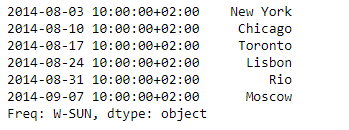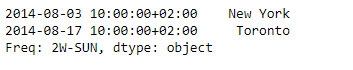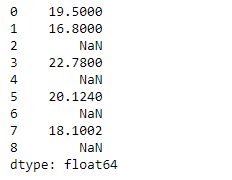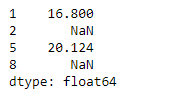# Python | Pandas Series.take()

• Last Updated : 05 Feb, 2019

Pandas series is a One-dimensional ndarray with axis labels. The labels need not be unique but must be a hashable type. The object supports both integer- and label-based indexing and provides a host of methods for performing operations involving the index.

Pandas` Series.take()` function return the elements in the given positional indices along an axis. Here we are not indexing according to actual values in the index attribute of the object. We are indexing according to the actual position of the element in the object.

Syntax: Series.take(indices, axis=0, convert=None, is_copy=True, **kwargs)

Parameter :
indices : An array of ints indicating which positions to take.
axis : The axis on which to select elements.
-> 0 means that we are selecting rows.
-> 1 means that we are selecting columns.
convert : Whether to convert negative indices into positive ones
is_copy : Whether to return a copy of the original object or not.
**kwargs : For compatibility with numpy.take(). Has no effect on the output.

Returns : taken : same type as caller

Example #1: Use `Series.take()` function to extract some elements from the given series object based on the actual positions of the elements in the object.

 `# importing pandas as pd``import` `pandas as pd`` ` `# Creating the Series``sr ``=` `pd.Series([``'New York'``, ``'Chicago'``, ``'Toronto'``, ``'Lisbon'``, ``'Rio'``, ``'Moscow'``])`` ` `# Create the Datetime Index``didx ``=` `pd.DatetimeIndex(start ``=``'2014-08-01 10:00'``, freq ``=``'W'``, ``                     ``periods ``=` `6``, tz ``=` `'Europe/Berlin'``) `` ` `# set the index``sr.index ``=` `didx`` ` `# Print the series``print``(sr)`

Output :Now we will use `Series.take()` function to extract values corresponding the passed positions.

 `# return elements corresponding to``# the passed index position``sr.take(indices ``=` `[``0``, ``2``])`

Output :As we can see in the output, `Series.take()` function has successfully returned the elements corresponding to the passed index positions of the given series object.

Example #2: Use `Series.take()` function to extract some elements from the given series object based on the actual positions of the elements in the object.

 `# importing pandas as pd``import` `pandas as pd`` ` `# Creating the Series``sr ``=` `pd.Series([``19.5``, ``16.8``, ``None``, ``22.78``, ``None``, ``20.124``, ``None``, ``18.1002``, ``None``])`` ` `# Print the series``print``(sr)`

Output :Now we will use `Series.take()` function to extract values corresponding the passed positions.

 `# return elements corresponding to``# the passed index position``sr.take(indices ``=` `[``1``, ``2``, ``5``, ``8``])`

Output :As we can see in the output, `Series.take()` function has successfully returned the elements corresponding to the passed index positions of the given series object.

My Personal Notes arrow_drop_up